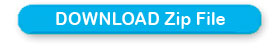# Difference in days between two dates of a calendar

We will develop a script to find the difference in two dates. We will return the difference in number of days. The input dates we will take from a calendar which we have already developed in date picker tutorials.

Read the tutorial on how to create date pickers

Our date inputs are available in two text boxes. Now on click of a button we will execute the function show_difference().
``<input type=button value='Find Difference' onClick='show_difference()';>``
Inside the function first we will read the date value of 1st input box.
``var t1=document.getElementById('t1').value``
We will use split string function to generate an array from the date input value.
``t1=t1.split('-');``
Now in our array the first element is month, 2nd element is date, 3rd element is year. So using this we can crate our date object.
``dt_t1=new Date(t1,t1,t1); // YYYY,mm,dd format to create date object``
Now using the same date object we will the millisecond value ( timestamp ) by using getTime() method.
``dt_t1_tm=dt_t1.getTime(); // time in milliseconds for day 1``
Same process is to be repeated for second date input box ( 2nd date value )
``````var t2=document.getElementById('t2').value
t2=t2.split('-');
dt_t2=new Date(t2,t2,t2);
dt_t2_tm=dt_t2.getTime();  time in milliseconds for day 2
``````
How many milliseconds are there in one day? The answer is here
``var one_day = 24*60*60*1000; // hours*minutes*seconds*milliseconds``
We can directly using the value also ( as : 86400000 )

Now we will divide the difference in milliseconds of both dates by the variable one_day.
``var diff_days=Math.abs((dt_t1_tm-dt_t2_tm)/one_day) // difference in days ``
We will take the Math floor value of the division as we would like to display 4.75 as 4 days. ( or you can use Math round )
``diff_days=Math.floor(diff_days);  // round off the difference in days to lower value``
Finally we will display the result in a div layer.
``````document.getElementById('display').innerHTML=' Difference in Days = ' + diff_days ;
document.getElementById('display').style.display = 'inline';
``````

difference of two dates taken from Calendar

Here is the soure of the function
``````function show_difference(){
var t1=document.getElementById('t1').value
t1=t1.split('-');
var dt_t1=new Date(t1,t1,t1); // YYYY,mm,dd format to create date object
var dt_t1_tm=dt_t1.getTime(); // time in milliseconds for day 1

var t2=document.getElementById('t2').value
t2=t2.split('-');
var dt_t2=new Date(t2,t2,t2);
var dt_t2_tm=dt_t2.getTime(); // time in milliseconds for day 2

var one_day = 24*60*60*1000; // hours*minutes*seconds*milliseconds
var diff_days=Math.abs((dt_t1_tm-dt_t2_tm)/one_day); // difference in days
diff_days=Math.floor(diff_days);  // round off the difference in days to lower value

/// Now display the result ///
document.getElementById('display').innerHTML=' Difference in Days = ' + diff_days ;
document.getElementById('display').style.display = 'inline';
}
``````Subscribe to our YouTube Channel here

## Subscribe

* indicates required
Subscribe to plus2netplus2net.com

More Projects on JavaScript using date object

Post your comments , suggestion , error , requirements etc here .